# Descriptive Epidemiology using epiR

#### 2020-03-13

Epidemiology is the study of the frequency, distribution and determinants of health-related states in populations and the application of such knowledge to control health problems (Centers for Disease Control and Prevention 2006).

This vignette provides instruction on the way R and epiR can be used for descriptive epidemiological analyses, that is, to describe how the frequency of disease varies by individual, place and time.

## Indivdual

Descriptions of disease frequency involves reporting either the prevalence or incidence of disease.

Some definitions. Strictly speaking, ‘prevalence’ equals the number of cases of a given disease or attribute that exists in a population at a specified point in time. Prevalence risk is the proportion of a population that has a specific disease or attribute at a specified point in time. Many authors use the term ‘prevalence’ when they really mean prevalence risk, and these notes will follow this convention.

Two types of prevalence are reported in the literature: (1) point prevalence equals the proportion of a population in a diseased state at a single point in time, (2) period prevalence equals the proportion of a population with a given disease or condition over a specific period of time (i.e. the number of existing cases at the start of a follow-up period plus the number of incident cases that occur during the follow-up period).

Incidence provides a measure of how frequently susceptible individuals become disease cases as they are observed over time. An incident case occurs when an individual changes from being susceptible to being diseased. The count of incident cases is the number of such events that occur in a population during a defined follow-up period. There are two ways to express incidence:

Incidence risk (also known as cumulative incidence) is the proportion of initially susceptible individuals in a population who become new cases during a defined follow-up period.

Incidence rate (also known as incidence density) is the number of new cases of disease that occur per unit of individual time at risk during a defined follow-up period.

In addition to reporting the point estimate of disease frequency, it is important to provide an indication of the uncertainty around that point estimate. The epi.conf function in the epiR package allows you to calculate confidence intervals for prevalence, incidence risk and incidence rates.

Let’s say we’re interested in the prevalence of disease X in a population comprised of 1000 individuals. Two hundred are tested and four returned a positive result. Assuming 100% test sensitivity and specificity, what is the estimated prevalence of disease X in this population?

library(epiR)

ncas <- 4; npop <- 200
tmp <- as.matrix(cbind(ncas, npop))
epi.conf(tmp, ctype = "prevalence", method = "exact", N = 1000, design = 1,
conf.level = 0.95) * 100
#>      est     lower    upper
#> ncas   2 0.5475566 5.041361

The estimated prevalence of disease X in this population is 2.0 (95% confidence interval [CI] 0.54 – 5.0) cases per 100 individuals at risk.

Another example. A study was conducted by Feychting, Osterlund, and Ahlbom (1998) to report the frequency of cancer among the blind. A total of 136 diagnoses of cancer were made from 22,050 person-years at risk. What was the incidence rate of cancer in this population?

ncas <- 136; ntar <- 22050
tmp <- as.matrix(cbind(ncas, ntar))
epi.conf(tmp, ctype = "inc.rate", method = "exact", N = 1000, design = 1,
conf.level = 0.95) * 1000
#>         est    lower    upper
#> ncas 6.1678 5.174806 7.295817

The incidence rate of cancer in this population was 6.2 (95% CI 5.2 to 7.3) cases per 1000 person-years at risk.

Now lets say we want to compare the frequency of disease across several populations. An effective way to do this is to used a ranked error bar plot. With a ranked error bar plot the points represent the point estimate of the measure of disease frequency and the error bars indicate the 95% confidence interval around each estimate. The disease frequency estimates are then sorted from lowest to highest.

Generate some data. First we’ll generate a distribution of disease prevalence estimates. Let’s say it has a mode of 0.60 and we’re 80% certain that the prevalence is greater than 0.35. Use the epi.betabuster function to generate shape1 and shape2 parameters that can be used for a beta distribution to satisfy these constraints:

tmp <- epi.betabuster(mode = 0.60, conf = 0.80, greaterthan = TRUE, x = 0.35,
conf.level = 0.95, max.shape1 = 100, step = 0.001)
tmp$shape1; tmp$shape2
#>  2.357
#>  1.904667

Take 100 draws from a beta distribution using the shape1 and shape2 values calculated above and plot them as a frequency histogram:


library(ggplot2)

dprob <- rbeta(n = 100, shape1 = tmp$shape1, shape2 = tmp$shape2)
dat <- data.frame(dprob = dprob)

ggplot(data = dat, aes(x = dprob)) +
geom_histogram(binwidth = 0.01, colour = "gray", size = 0.1) +
scale_x_continuous(limits = c(0,1), name = "Prevalence") +
scale_y_continuous(limits = c(0,10), name = "Number of draws")
#> Warning: Removed 2 rows containing missing values (geom_bar).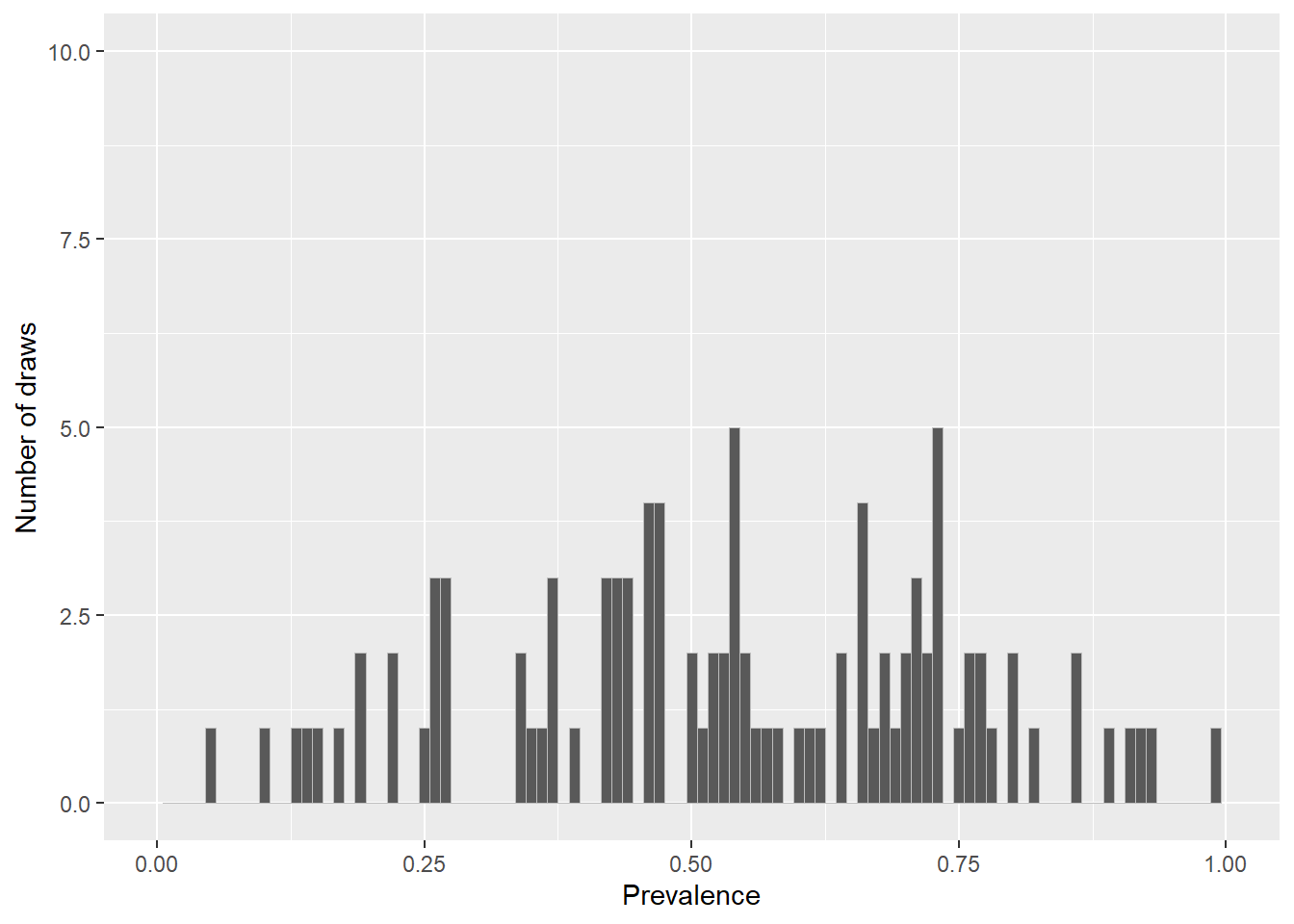Frequency histogram of disease prevalence estimates for our simulated population.

Generate a vector of population sizes using the uniform distribution. Calculate the number of diseased individuals in each population using dprob (calculated above). Finally, calculate the prevalence of disease in each population and its 95% confidence interval using epi.conf. The function epi.conf provides several options for confidence interval calculation method for prevalence. Here we’ll use the exact method:

dat$npop <- round(runif(n = 100, min = 20, max = 1500), digits = 0) dat$ncas <- round(dat$dprob * dat$npop, digits = 0)

tmp <- as.matrix(cbind(dat$ncas, dat$npop))
tmp <- epi.conf(tmp, ctype = "prevalence", method = "exact", N = 1000, design = 1,
conf.level = 0.95) * 100
dat <- cbind(dat, tmp)
#>       dprob npop ncas      est    lower    upper
#> 1 0.5401015  127   69 54.33071 45.26106 63.19379
#> 2 0.4990490 1004  501 49.90040 46.76197 53.03941
#> 3 0.3901477  728  284 39.01099 35.44977 42.66163
#> 4 0.7273786  669  487 72.79522 69.25261 76.13530
#> 5 0.9319481 1276 1189 93.18182 91.65752 94.50321
#> 6 0.7689964  881  677 76.84449 73.91508 79.59311

Sort the data in order of variable est and assign a 1 to n identifier as variable rank:

dat <- dat[sort.list(dat$est),] dat$rank <- 1:nrow(dat)

Now create the ranked error bar plot:


ggplot(data = dat, aes(x = rank, y = est)) +
geom_errorbar(aes(ymin = lower, ymax = upper), width = 0.1) +
geom_point() +
scale_x_continuous(limits = c(0,100), name = "Rank") +
scale_y_continuous(limits = c(0,100), name = "Prevalence (cases per 100 individuals
at risk)")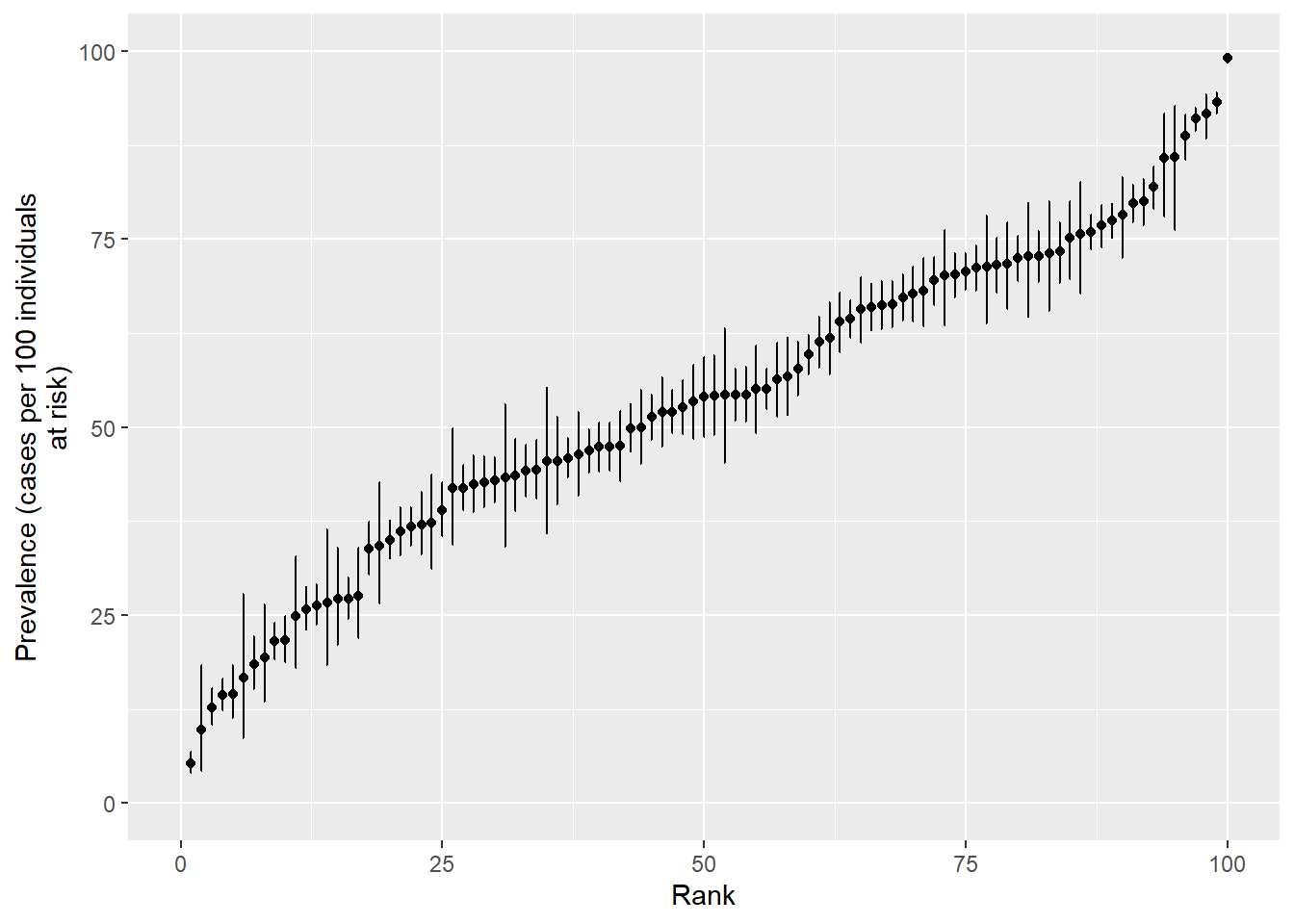Ranked error bar plot showing the prevalence of disease (and its 95% confidence interval) for 100 population units.

## Time

Epidemic curve data are often presented in one of two formats:

1. One row for each individual identified as a case with an event date assigned to each.

2. One row for every event date with an integer representing the number of cases identified on that date.

We first generate some data, with one row for every individual identified as a case:

n.males <- 100; n.females <- 50
odate <- seq(from = as.Date("2004-07-26"), to = as.Date("2004-08-13"), by = 1)
prob <- c(1:10, 9:1); prob <- prob/sum(prob)
modate <- sample(x = odate, size = n.males, replace = TRUE, p = prob)
fodate <- sample(x = odate, size = n.females, replace = TRUE)
dat <- data.frame(sex = c(rep("Male", n.males), rep("Female", n.females)),
odate = c(modate, fodate))

Plot the epidemic curve using the ggplot2 and scales packages:


library(ggplot2); library(scales)

ggplot(data = dat, aes(x = as.Date(odate))) +
geom_histogram(binwidth = 1, colour = "gray", size = 0.1) +
scale_x_date(breaks = date_breaks("1 week"), labels = date_format("%d %b"),
name = "Date") +
scale_y_continuous(limits = c(0, 30), name = "Number of cases") +
theme(axis.text.x = element_text(angle = 90, hjust = 1))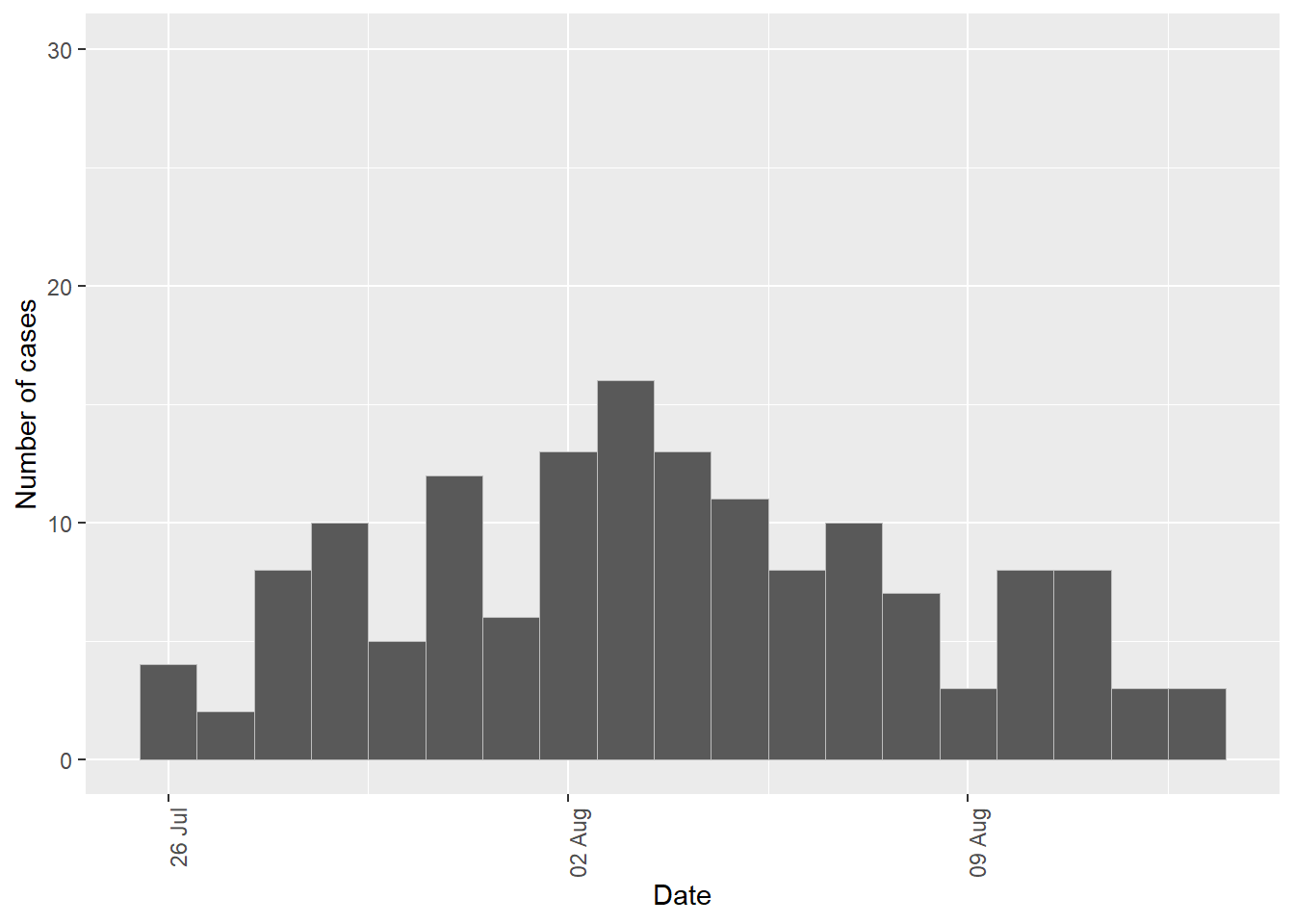Frequency histogram showing counts of incident cases of disease as a function of time, 26 July to 13 August 2004.

Produce a separate epidemic curve for males and females using the facet_grid option in ggplot2:


ggplot(data = dat, aes(x = as.Date(odate))) +
geom_histogram(binwidth = 1, colour = "gray", size = 0.1) +
scale_x_date(breaks = date_breaks("1 week"), labels = date_format("%d %b"),
name = "Date") +
scale_y_continuous(limits = c(0, 30), name = "Number of cases") +
theme(axis.text.x = element_text(angle = 90, hjust = 1)) +
facet_grid( ~ sex)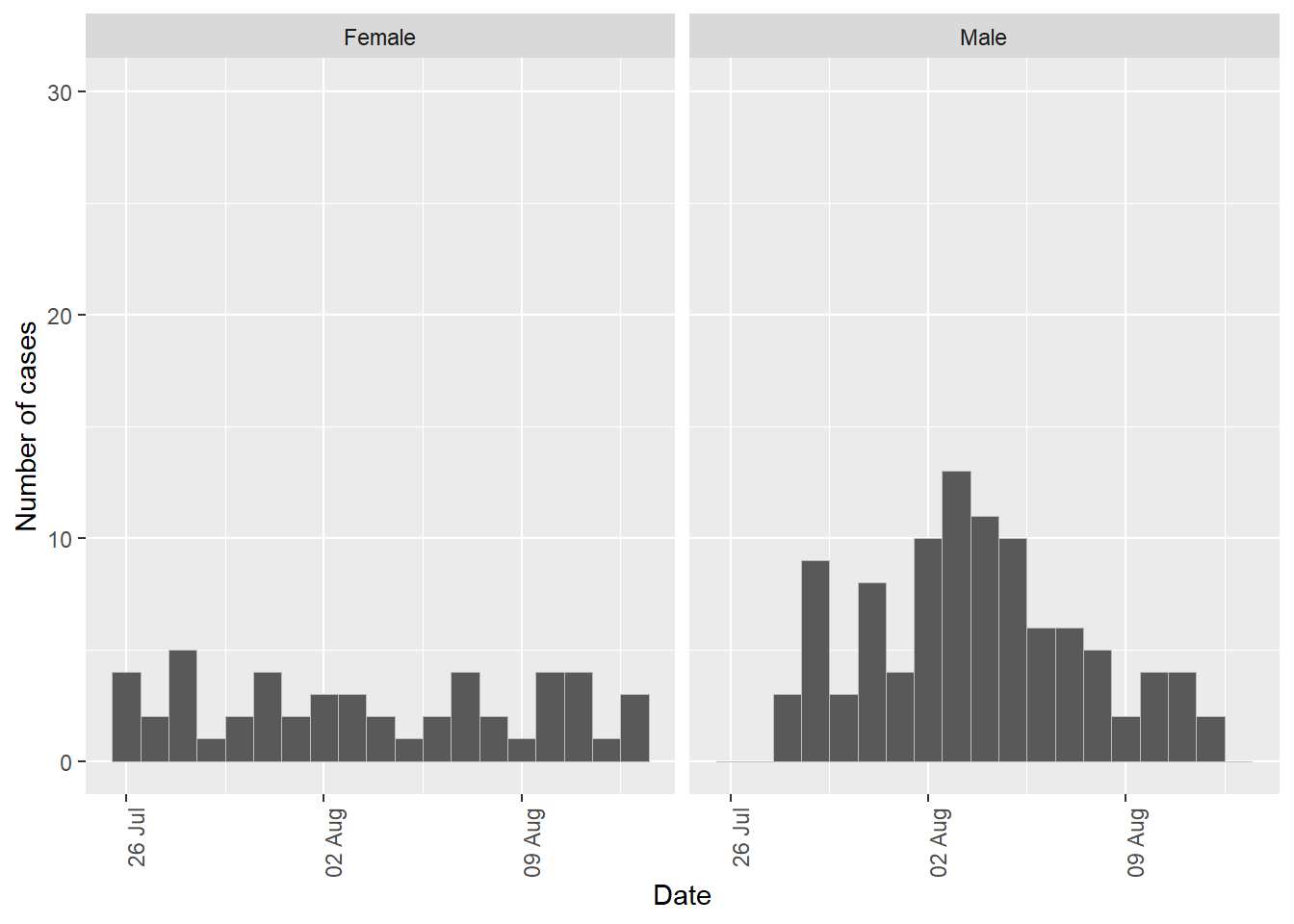Frequency histogram showing counts of incident cases of disease as a function of time, 26 July to 13 August 2004, conditioned by sex.

Let’s say some event occurred on 31 July 2003. Mark this date on your epidemic curve using geom_vline:


ggplot(data = dat, aes(x = as.Date(odate))) +
geom_histogram(binwidth = 1, colour = "gray", size = 0.1) +
scale_x_date(breaks = date_breaks("1 week"), labels = date_format("%d %b"),
name = "Date") +
scale_y_continuous(limits = c(0, 30), name = "Number of cases") +
theme(axis.text.x = element_text(angle = 90, hjust = 1)) +
facet_grid( ~ sex) +
geom_vline(aes(xintercept = as.numeric(as.Date("31/07/2004", format = "%d/%m/%Y"))),
linetype = "dashed")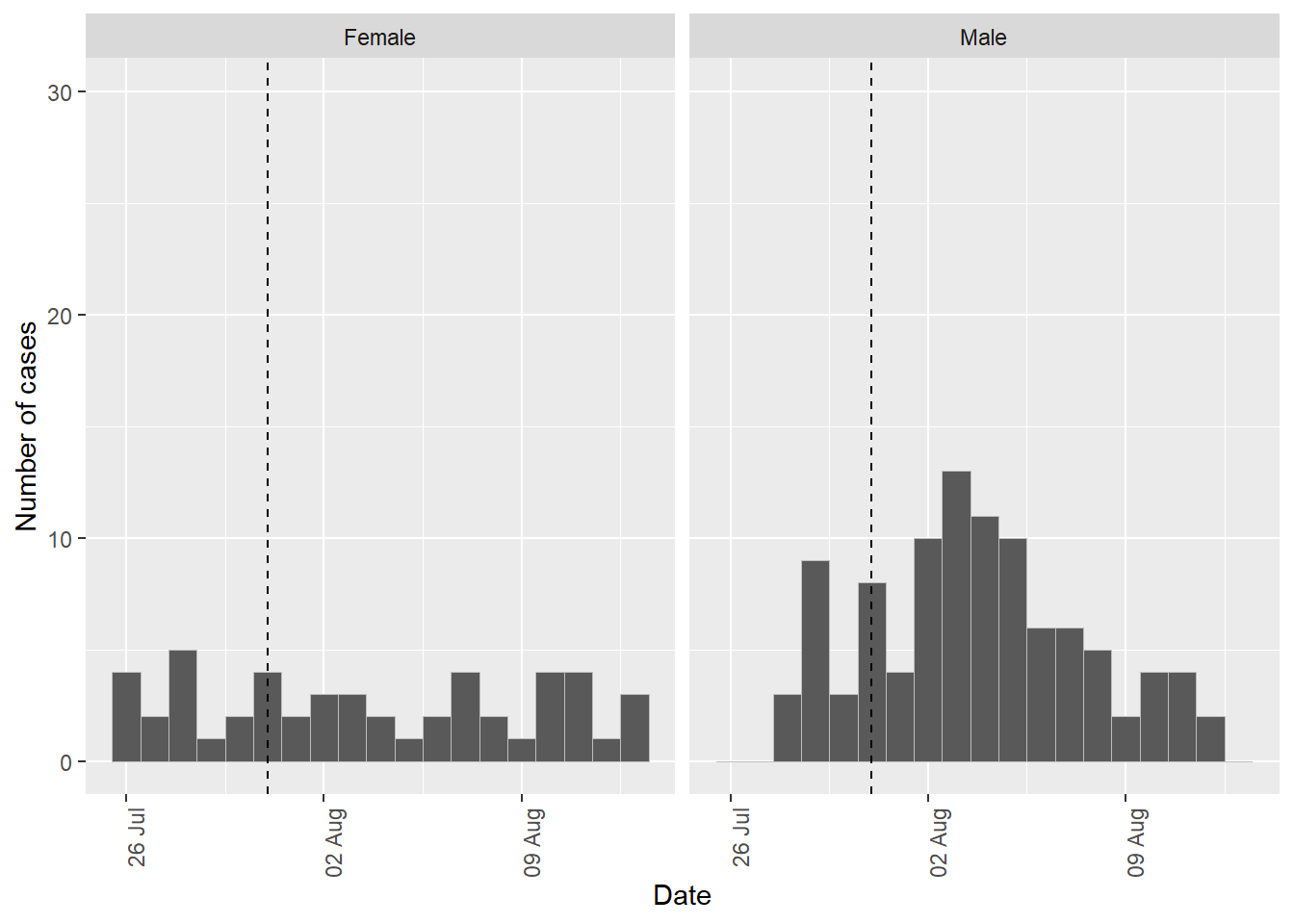Frequency histogram showing counts of incident cases of disease as a function of time, 26 July to 13 August 2004, conditioned by sex. An important event that occurred on 31 July 2004 is indicated by the vertical dashed line.

Plot the total number of disease events by day, coloured according to sex:


ggplot(data = dat, aes(x = as.Date(odate), group = sex, fill = sex)) +
geom_histogram(binwidth = 1, colour = "gray", size = 0.1) +
scale_x_date(breaks = date_breaks("1 week"), labels = date_format("%d %b"),
name = "Date") +
scale_y_continuous(limits = c(0, 30), name = "Number of cases") +
theme(axis.text.x = element_text(angle = 90, hjust = 1)) +
geom_vline(aes(xintercept = as.numeric(as.Date("31/07/2004", format = "%d/%m/%Y"))),
linetype = "dashed") +
scale_fill_manual(values = c("#d46a6a", "#738ca6"), name = "Sex") +
theme(legend.position = c(0.90, 0.80))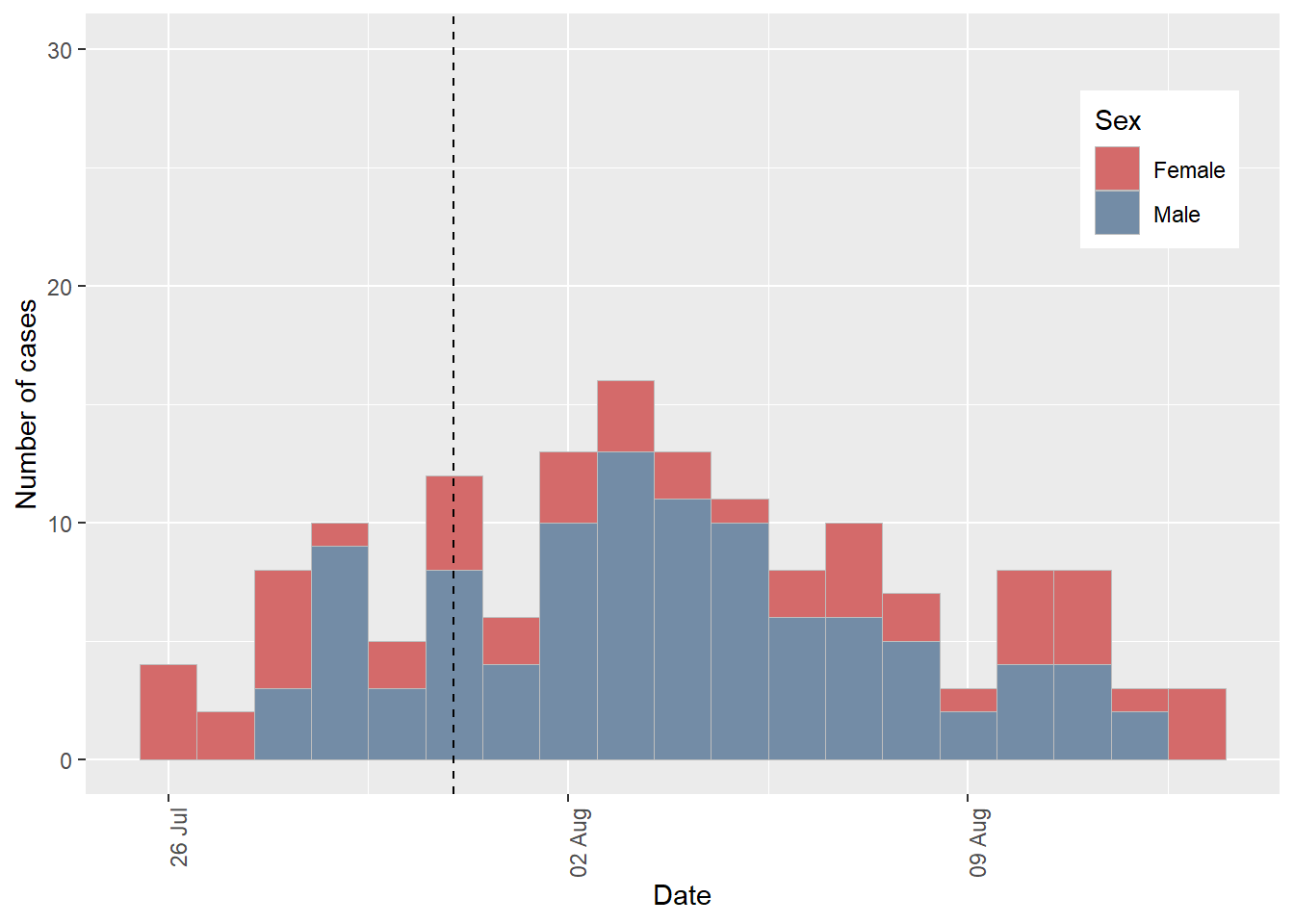Frequency histogram showing counts of incident cases of disease as a function of time, 26 July to 13 August 2004, grouped by sex.

It can be difficult to appreciate differences in male and female disease counts as a function of date with the above plot format so we dodge the data instead.


ggplot(data = dat, aes(x = as.Date(odate), group = sex, fill = sex)) +
geom_histogram(binwidth = 1, colour = "gray", size = 0.1, position = "dodge") +
scale_x_date(breaks = date_breaks("1 week"), labels = date_format("%d %b"),
name = "Date") +
scale_y_continuous(limits = c(0, 30), name = "Number of cases") +
theme(axis.text.x = element_text(angle = 90, hjust = 1)) +
geom_vline(aes(xintercept = as.numeric(as.Date("31/07/2004", format = "%d/%m/%Y"))),
linetype = "dashed") +
scale_fill_manual(values = c("#d46a6a", "#738ca6"), name = "Sex") +
theme(legend.position = c(0.90, 0.80))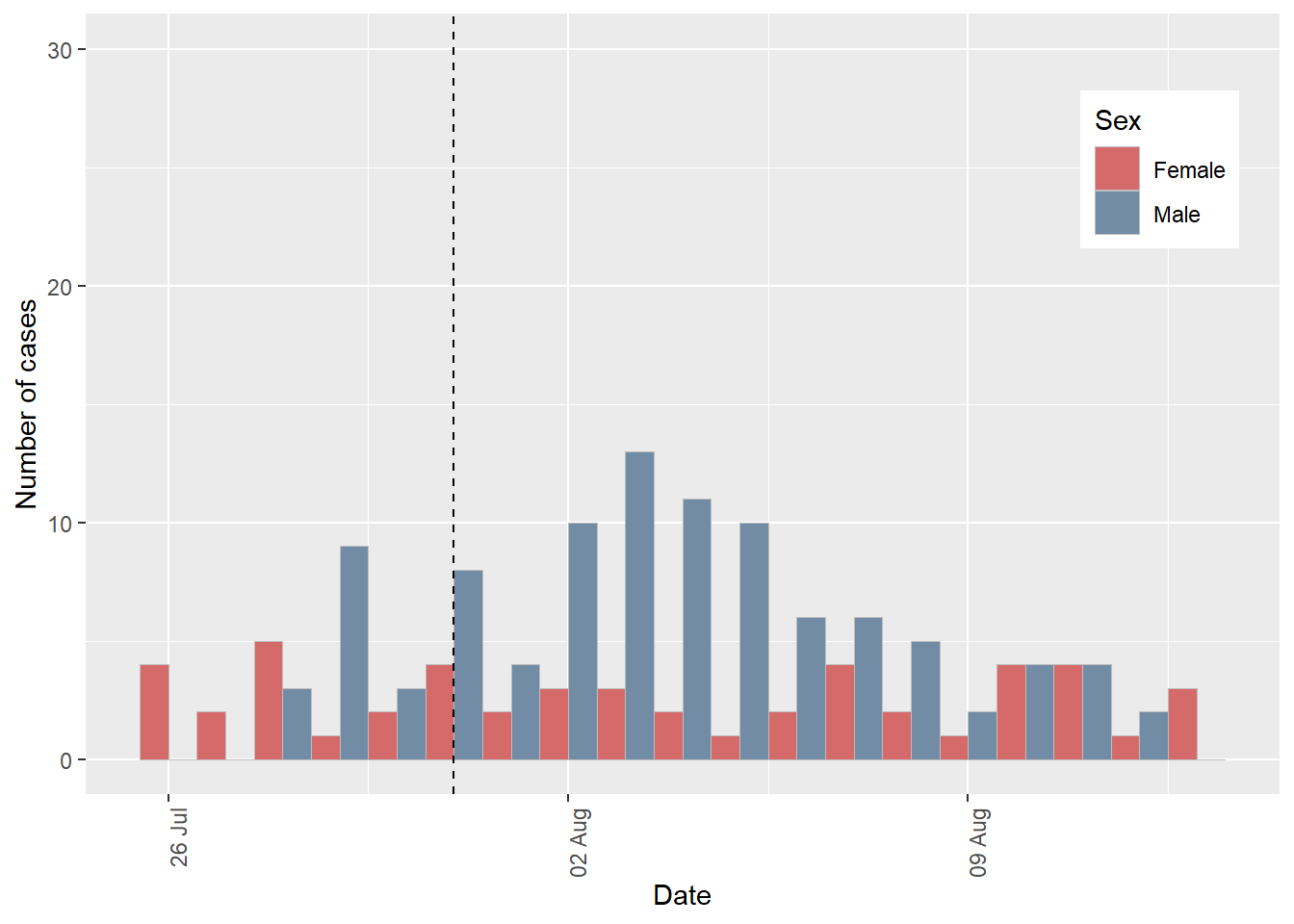Frequency histogram showing counts of incident cases of disease as a function of time, 26 July to 13 August 2004, grouped by sex.

We now provide code to deal with the situation where the data are presented with one row for every case event date and an integer representing the number of cases identified on each date.

Simulate some data in this format. In the code below the variable ncas represents the number of cases identified on a given date. The variable dcontrol is a factor with two levels: neg and pos. Level neg flags dates when no disease control measures were in place; level pos flags dates when disease controls measures were in place.

odate <- seq(from = as.Date("1/1/00", format = "%d/%m/%y"),
to = as.Date("1/1/05", format = "%d/%m/%y"), by = "1 month")
ncas <- round(runif(n = length(odate), min = 0, max = 100), digits = 0)
dat <- data.frame(odate, ncas)
dat$dcontrol <- "neg" dat$dcontrol[dat$odate >= as.Date("1/1/03", format = "%d/%m/%y") & dat$odate <= as.Date("1/6/03", format = "%d/%m/%y")] <- "pos"
#>        odate ncas dcontrol
#> 1 2000-01-01   32      neg
#> 2 2000-02-01   21      neg
#> 3 2000-03-01   26      neg
#> 4 2000-04-01   31      neg
#> 5 2000-05-01   76      neg
#> 6 2000-06-01   21      neg

Generate an epidemic curve. Note weight = ncas in the aesthetics argument for ggplot2:


ggplot(dat, aes(x = odate, weight = ncas, fill = factor(dcontrol))) +
geom_histogram(binwidth = 60, colour = "gray", size = 0.1) +
scale_x_date(breaks = date_breaks("6 months"), labels = date_format("%b %Y"),
name = "Date") +
scale_y_continuous(limits = c(0, 200), name = "Number of cases") +
scale_fill_manual(values = c("#2f4f4f", "red")) +
guides(fill = FALSE) +
theme(axis.text.x = element_text(angle = 90, hjust = 1))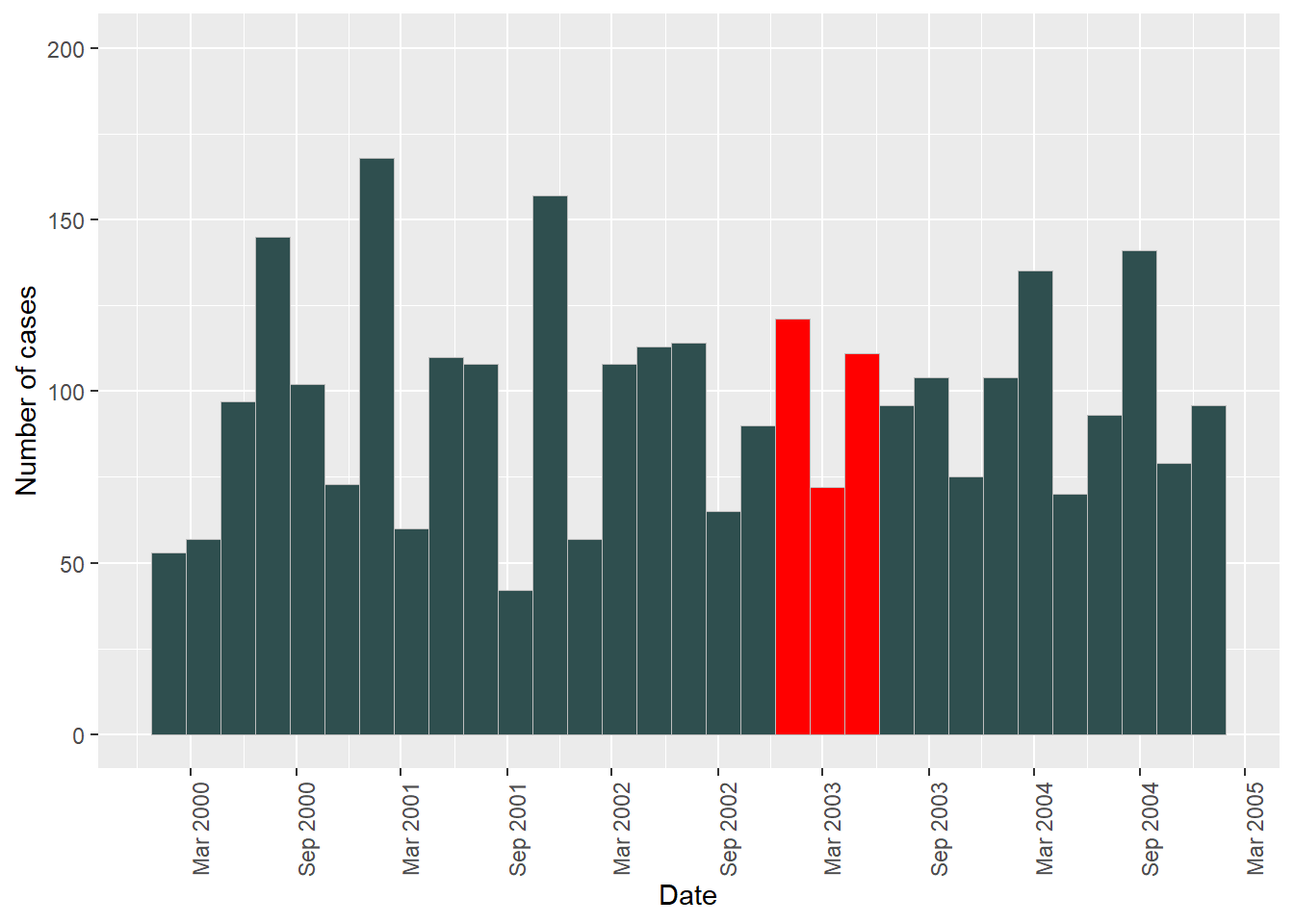Frequency histogram showing counts of incident cases of disease as a function of time, 1 January 2000 to 1 January 2005. Colours indicate the presence or absence of disease control measures.

## Place

Two types of maps are often used when describing patterns of disease by place:

1. Choropleth maps. Choropleth mapping involves producing a summary statistic of the outcome of interest (e.g. count of disease events, prevalence, incidence) for each component area within a study region. A map is created by ‘filling’ (i.e. colouring) each component area with colour, providing an indication of the magnitude of the variable of interest and how it varies geographically.

2. Point maps.

Choropleth maps

For illustration we make a choropleth map of sudden infant death syndrome (SIDS) babies in North Carolina counties for 1974 using the nc.sids data provided with the spData package.

library(spData); library(rgeos); library(rgdal); library(plyr); library(RColorBrewer)

ncsids.shp <- readOGR(system.file("shapes/sids.shp", package = "spData"))
#> OGR data source with driver: ESRI Shapefile
#> Source: "C:\Program Files\R\R-3.6.3\library\spData\shapes\sids.shp", layer: "sids"
#> with 100 features
#> It has 22 fields
ncsids.shp@data <- ncsids.shp@data[,c("BIR74","SID74")]
#>   BIR74 SID74
#> 0  1091     1
#> 1   487     0
#> 2  3188     5
#> 3   508     1
#> 4  1421     9
#> 5  1452     7

The ncsids.shp spatialPolygonsDataframe lists for each county in the North Carolina USA the number SIDS deaths for 1974.

Prepare the spatialPolygonsDataframe by creating a 1 to n identifier called id. We then fortify the spatialPolygonsDataframe to allow it to be used with ggplot2. Finally, join the attribute data from spatialPolygonsDataframe ncsids.shp to the fortified ncsids.df, using variable id as the key:

ncsids.shp$id <- 1:nrow(ncsids.shp@data) ncsids.df <- fortify(ncsids.shp, region = "id") ncsids.df <- join(x = ncsids.df, y = ncsids.shp@data, by = "id") Choropleth map of the counties of the North Carolina showing SIDS counts for 1974:  ggplot(data = ncsids.df) + theme_bw() + geom_polygon(aes(x = long, y = lat, group = group, fill = SID74)) + geom_path(aes(x = long, y = lat, group = group), colour = "grey", size = 0.25) + scale_fill_gradientn(limits = c(0, 60), colours = brewer.pal(n = 5, "Reds"), guide = "colourbar") + scale_x_continuous(name = "Longitude") + scale_y_continuous(name = "Latitude") + labs(fill = "SIDS 1974") + coord_map()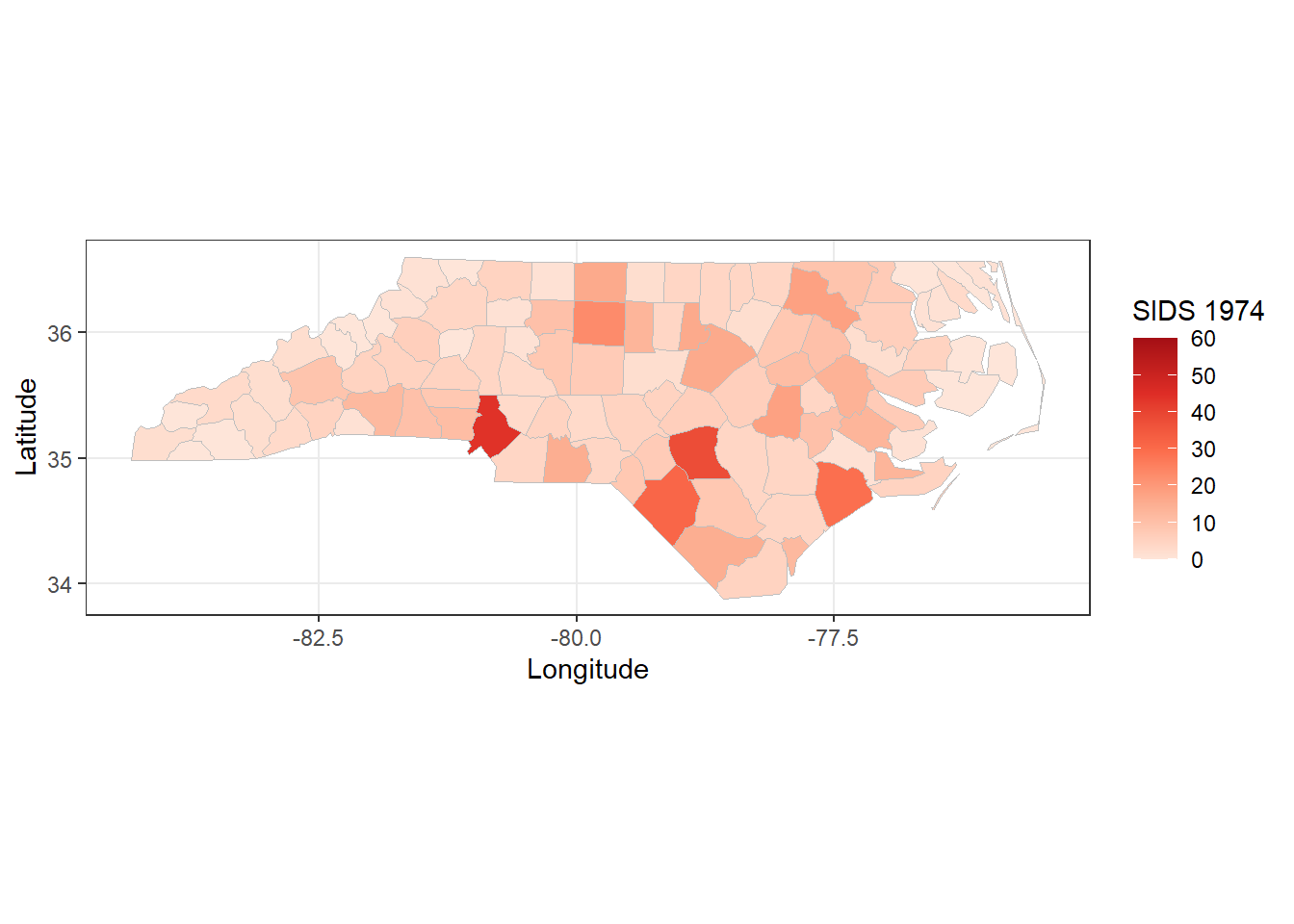Map of North Carolina, USA showing the number of sudden infant death syndrome cases, by county for 1974. Point maps For this example we will used the epi.incin data set included with epiR. Between 1972 and 1980 an industrial waste incinerator operated at a site about 2 kilometres southwest of the town of Coppull in Lancashire, England. Addressing community concerns that there were greater than expected numbers of laryngeal cancer cases in close proximity to the incinerator Diggle (1990) conducted a study investigating risks for laryngeal cancer, using recorded cases of lung cancer as controls. The study area is 20 km x 20 km in size and includes location of residence of patients diagnosed with each cancer type from 1974 to 1983. Load the epi.incin data set and create negative and positive labels for each point location. We don’t have a boundary map for these data so we’ll use spatstat to create a convex hull around the points and dilate the convex hull by 1000 metres as a proxy boundary. Create an observation window for the data as dat.w and a ppp object for plotting: library(spatstat) data(epi.incin); dat.df <- epi.incin dat.df$status <- factor(dat.df$status, levels = c(0,1), labels = c("Neg", "Pos")) names(dat.df) <- "Status" dat.w <- convexhull.xy(x = dat.df[,1], y = dat.df[,2]) dat.w <- dilation(dat.w, r = 1000) dat.ppp <- ppp(x = dat.df[,1], y = dat.df[,2], marks = factor(dat.df[,3]), window = dat.w) Create a SpatialPolygonsDataFrame from dat.w: coords <- matrix(c(dat.w$bdry[]$x, dat.w$bdry[]$y), ncol = 2, byrow = FALSE) pol <- Polygon(coords, hole = FALSE) pol <- Polygons(list(pol),1) pol <- SpatialPolygons(list(pol)) pol.spdf <- SpatialPolygonsDataFrame(Sr = pol, data = data.frame(id = 1), match.ID = TRUE) pol.map <- fortify(pol.spdf) #> Regions defined for each Polygons Plot the data as a point map:  ggplot() + geom_point(data = dat.df, aes(x = xcoord, y = ycoord, colour = Status, shape = Status)) + geom_polygon(data = pol.map, aes(x = long, y = lat, group = group), col = "black", fill = "transparent") + scale_colour_manual(values = c("blue", "red")) + scale_shape_manual(values = c(1,16)) + labs(x = "Easting (m)", y = "Northing (m)", fill = "Status") + coord_equal() + theme_bw()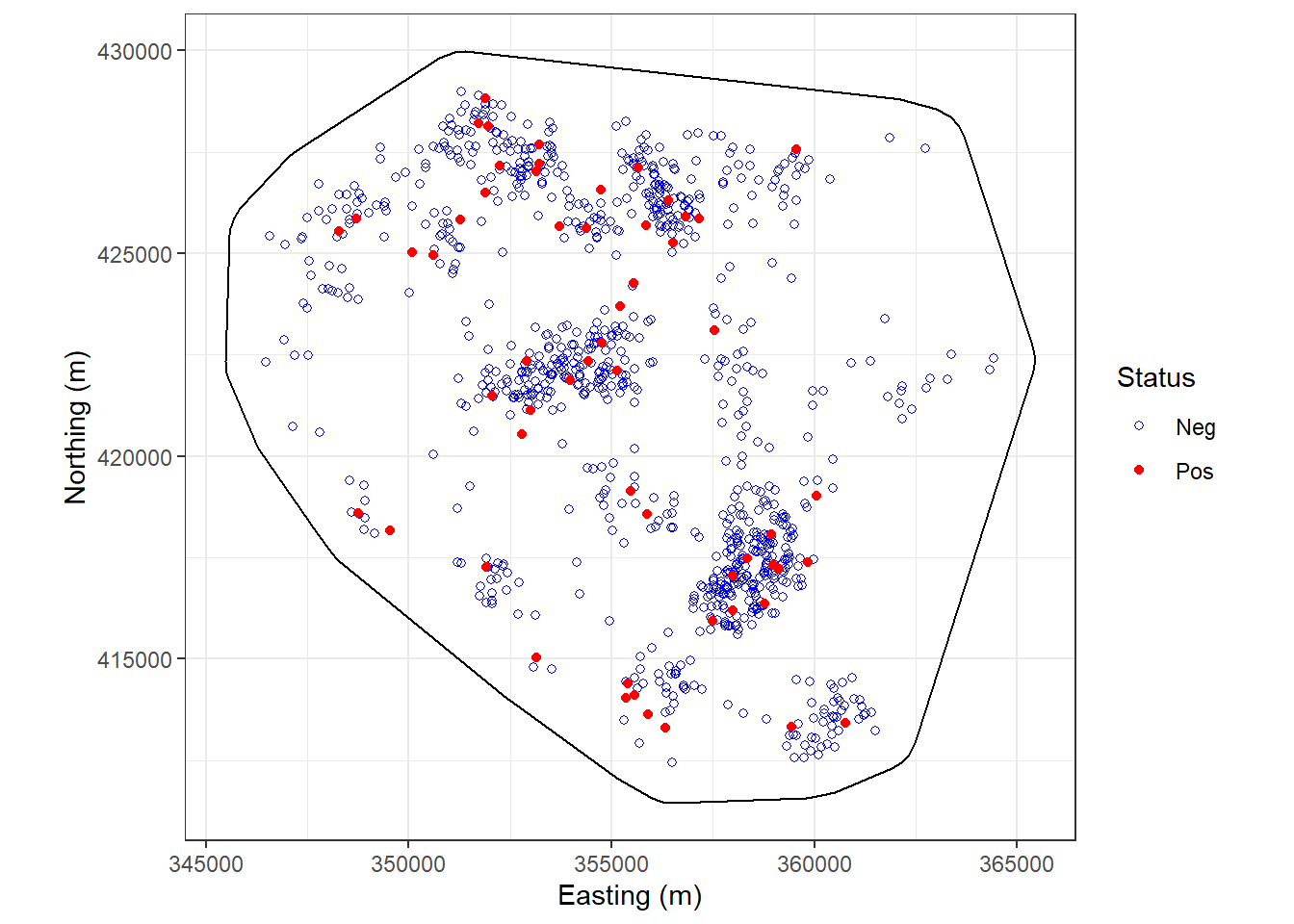Point map showing the place of residence of individuals diagnosed with laryngeal cancer (Pos) and lung cancer (Neg), Copull Lancashire, UK, 1972 to 1980. ## Measures of association An important task in epidemiology is to quantify the strength of association between exposures and outcomes. In this context the term ‘exposure’ is taken to mean a variable whose association with the outcome is to be estimated. Exposures can be harmful, beneficial or both harmful and beneficial (e.g. if an immunisable disease is circulating, exposure to immunising agents helps most recipients but may harm those who experience adverse reactions). The term outcome’ is used to describe all the possible results that may arise from exposure to a causal factor or from preventive or therapeutic interventions (Porta, Greenland, and Last 2008). In this section we outline describe how epiR can be used to compute the various measures of association used in epidemiology notably the risk ratio, odds ratio, attributable risk, attributable fraction, population attributable risk and population attributable fraction. Examples are provided to demonstrate how the package can be used to deal with exposure-outcome data in various formats. Some preliminary comments. The epi.2by2 function in epiR requires an object of class table as input. This vignette has been written assuming the reader routinely formats their 2 by 2 table data with the outcome status as columns and exposure status as rows. If this is not the case the argument outcome = "as.columns" (the default) can be changed to outcome = "as.rows". Direct entry of cell frequencies Create a 2 by 2 table by keying in the cell frequencies. A cross sectional study investigating the relationship between dry cat food (DCF) and feline lower urinary tract disease (FLUTD) was conducted (Willeberg 1977). Counts of individuals in each group were as follows. DCF-exposed cats (cases, non-cases) 13, 2163. Non DCF-exposed cats (cases, non-cases) 5, 3349. Enter these data directly into R as a matrix: flutd.tab <- matrix(c(13,2163,5,3349), nrow = 2, byrow = TRUE) rownames(flutd.tab) <- c("DF+", "DF-"); colnames(flutd.tab) <- c("FLUTD+", "FLUTD-") flutd.tab <- as.table(flutd.tab); flutd.tab #> FLUTD+ FLUTD- #> DF+ 13 2163 #> DF- 5 3349 Calculate the prevalence ratio, odds ratio, attributable prevalence, the attributable prevalence in the population, the attributable fraction in the exposed and the attributable fraction in the population using epi.2by2: epi.2by2(dat = flutd.tab, method = "cross.sectional", conf.level = 0.95, units = 100, outcome = "as.columns") #> Outcome + Outcome - Total Prevalence * Odds #> Exposed + 13 2163 2176 0.597 0.00601 #> Exposed - 5 3349 3354 0.149 0.00149 #> Total 18 5512 5530 0.325 0.00327 #> #> Point estimates and 95% CIs: #> ------------------------------------------------------------------- #> Prevalence ratio 4.01 (1.43, 11.23) #> Odds ratio 4.03 (1.43, 11.31) #> Attrib prevalence * 0.45 (0.10, 0.80) #> Attrib prevalence in population * 0.18 (-0.02, 0.38) #> Attrib fraction in exposed (%) 75.05 (30.11, 91.09) #> Attrib fraction in population (%) 54.20 (3.61, 78.24) #> ------------------------------------------------------------------- #> Test that OR = 1: chi2(1) = 8.177 Pr>chi2 = 0.00 #> Wald confidence limits #> CI: confidence interval #> * Outcomes per 100 population units The prevalence of FLUTD in DCF exposed cats was 4.01 (95% CI 1.43 to 11.23) times greater than the prevalence of FLUTD in non-DCF exposed cats. In DCF exposed cats, 75% of FLUTD was attributable to DCF (95% CI 30% to 91%). Fifty-four percent of FLUTD cases in this cat population were attributable to DCF (95% CI 4% to 78%). Data frame with one row per observation For this example we use the low infant birth weight data presented by Hosmer and Lemeshow (2000) and available in the MASS package in R. The birthwt data frame has 189 rows and 10 columns. The data were collected at Baystate Medical Center, Springfield, Massachusetts USA during 1986. library(MASS) #> #> Attaching package: 'MASS' #> The following object is masked from 'package:spatstat': #> #> area bwt <- birthwt; head(bwt) #> low age lwt race smoke ptl ht ui ftv bwt #> 85 0 19 182 2 0 0 0 1 0 2523 #> 86 0 33 155 3 0 0 0 0 3 2551 #> 87 0 20 105 1 1 0 0 0 1 2557 #> 88 0 21 108 1 1 0 0 1 2 2594 #> 89 0 18 107 1 1 0 0 1 0 2600 #> 91 0 21 124 3 0 0 0 0 0 2622 Each row of this data set represents data for one mother. We’re interested in the association between smoke (the mother’s smoking status during pregnancy) and low (delivery of a baby less than 2.5 kg bodyweight). Its important that the table you present to epi.2by2 is in the correct format: Disease positives in the first column, disease negatives in the second column, exposure positives in the first row and exposure negatives in the second row. If we run the table function on the bwt data the output table is in the wrong format: low.tab <- table(bwt$smoke, bwt$low, dnn = c("Smoke", "Low BW")); low.tab #> Low BW #> Smoke 0 1 #> 0 86 29 #> 1 44 30 There are two approaches for fixing this problem. For the first aproach we ask R to switch the order of the rows and columns: low.tab <- table(bwt$smoke, bwt$low, dnn = c("Smoke", "Low BW")) low.tab <- low.tab[2:1,2:1]; low.tab #> Low BW #> Smoke 1 0 #> 1 30 44 #> 0 29 86 The second approach is to set the exposure variable and the outcome variable as a factor and to define the levels of each factor using levels = c(1,0): bwt$low <- factor(bwt$low, levels = c(1,0)) bwt$smoke <- factor(bwt$smoke, levels = c(1,0)) bwt$race <- factor(bwt$race, levels = c(1,2,3)) Now generate the 2 by 2 table. Exposure (rows) = smoke, outcome (columns) = low: low.tab <- table(bwt$smoke, bwt$low, dnn = c("Smoke", "Low BW")); low.tab #> Low BW #> Smoke 1 0 #> 1 30 44 #> 0 29 86 Compute the odds ratio for smoking and delivery of a low birth weight baby: epi.2by2(dat = low.tab, method = "cohort.count", conf.level = 0.95, units = 100, outcome = "as.columns") #> Outcome + Outcome - Total Inc risk * Odds #> Exposed + 30 44 74 40.5 0.682 #> Exposed - 29 86 115 25.2 0.337 #> Total 59 130 189 31.2 0.454 #> #> Point estimates and 95% CIs: #> ------------------------------------------------------------------- #> Inc risk ratio 1.61 (1.06, 2.44) #> Odds ratio 2.02 (1.08, 3.78) #> Attrib risk * 15.32 (1.61, 29.04) #> Attrib risk in population * 6.00 (-4.33, 16.33) #> Attrib fraction in exposed (%) 37.80 (5.47, 59.07) #> Attrib fraction in population (%) 19.22 (-0.21, 34.88) #> ------------------------------------------------------------------- #> Test that OR = 1: chi2(1) = 4.924 Pr>chi2 = 0.03 #> Wald confidence limits #> CI: confidence interval #> * Outcomes per 100 population units The odds of having a low birth weight child for smokers is 2.02 (95% CI 1.08 to 3.78) times greater than the odds of having a low birth weight child for non-smokers. We’re concerned that the mother’s race may confound the association between low birth weight and delivery of a low birth weight baby. Stratify the 2 by 2 table by race: low.stab <- table(bwt$smoke, bwt$low, bwt$race, dnn = c("Smoke", "Low BW", "Race"))
low.stab
#> , , Race = 1
#>
#>      Low BW
#> Smoke  1  0
#>     1 19 33
#>     0  4 40
#>
#> , , Race = 2
#>
#>      Low BW
#> Smoke  1  0
#>     1  6  4
#>     0  5 11
#>
#> , , Race = 3
#>
#>      Low BW
#> Smoke  1  0
#>     1  5  7
#>     0 20 35

Compute the crude odds ratio and the Mantel-Haenszel adjusted odds ratio. epi.2by2 automatically calculates the Mantel-Haenszel odds ratio and risk ratio when it is presented with stratified contingency tables.

rval <- epi.2by2(dat = low.stab, method = "cohort.count", conf.level = 0.95,
units = 100, outcome = "as.columns")
print(rval)
#>              Outcome +    Outcome -      Total        Inc risk *        Odds
#> Exposed +           30           44         74              40.5       0.682
#> Exposed -           29           86        115              25.2       0.337
#> Total               59          130        189              31.2       0.454
#>
#>
#> Point estimates and 95% CIs:
#> -------------------------------------------------------------------
#> Inc risk ratio (crude)                       1.61 (1.06, 2.44)
#> Inc risk ratio (M-H)                         2.15 (1.29, 3.58)
#> Inc risk ratio (crude:M-H)                   0.75
#> Odds ratio (crude)                           2.02 (1.08, 3.78)
#> Odds ratio (M-H)                             3.09 (1.49, 6.39)
#> Odds ratio (crude:M-H)                       0.66
#> Attrib risk (crude) *                        15.32 (1.61, 29.04)
#> Attrib risk (M-H) *                          22.17 (1.41, 42.94)
#> Attrib risk (crude:M-H)                      0.69
#> -------------------------------------------------------------------
#>  M-H test of homogeneity of RRs: chi2(2) = 3.862 Pr>chi2 = 0.15
#>  M-H test of homogeneity of ORs: chi2(2) = 2.800 Pr>chi2 = 0.25
#>  Test that M-H adjusted OR = 1:  chi2(2) = 9.413 Pr>chi2 = 0.00
#>  Wald confidence limits
#>  M-H: Mantel-Haenszel; CI: confidence interval
#>  * Outcomes per 100 population units`

The Mantel-Haenszel test of homogeneity of the strata odds ratios is not significant (chi square test statistic 2.800; df 2; p-value = 0.25) so we accept the null hypothesis and conclude that the odds ratios for each strata of race are the same. After accounting for the confounding effect of race, the odds of having a low birth weight child for smokers is 3.09 (95% CI 1.49 to 6.39) times that of non-smokers.